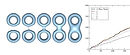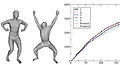PUBLICATIONS

Our publications provide and in-depth study of the various properties of the mesh Laplace operator when the underlying manifold (or surface) undergoes changes (like noise, resolution and even topological changes). They also introduce various applications, like matching partial and incomplete shapes, segmentation and mesh deformation for animation purposes. Click here to view my Google Scholar citation page.###### Custom Video Metrics Management Platform

US Patent Grant/Publication: 2018-09-04, Priority Date: 2014-12-08
Authors: Haijie Wu, Snehal Karia, Pawas Ranjan, Faizal Zakaria Siddiqi

Data collection management is disclosed. A data collection configuration is obtained. The data collection configuration is translated into executable code in a language usable to collect data. Data is collected using the executable code. The collected data values are provided as output. Metrics management is also disclosed. A configuration of a metric is obtained. The metric configuration includes a definition of how computation of the metric is to be performed and a mapping between a computation input and collected data. Collected data values are obtained based at least in part on the mapping. Metric values are computed according to the definition. One or more results associated with the computed metric values are stored.###### Smart Scaling of Unique-based metrics using HyperLogLog

Technical Blog for Conviva Inc 2015
Authors: Pawas Ranjan

At Conviva, we empower top media publishers by providing them with rich real-time insights on their video streaming traffic. We compute different Quality of Experience (QoE) metrics like Rebuffering Ratio, Average Bitrate, Video Startup Time and many more. A critical metric for our customers is the number of Unique Viewers (Daily/Monthly Unique), which happens to be one of the few metrics that cannot be directly added up. For example, we cannot simply add the number of unique viewers for each day of the week to get the number of unique viewers for the entire week.

The truly accurate way is to maintain the sets of unique ids for each day and then find the cardinality of the union of the sets. This is not feasible computationally or storage-wise, since we typically see millions of unique viewers each day. Instead, we decided to use the relatively recent HyperLogLog algorithm, which provides an estimation of the cardinality of a set and, more importantly, is ‘aggregatable’.###### Weighted Graph Laplace Operator under Topological Noise

Proceedings of the Twenty-Fourth Annual ACM-SIAM Symposium on Discrete Algorithms (SODA) 2013
Authors: Tamal K. Dey, Pawas Ranjan, Yusu Wang

Recently, various applications have motivated the study of spectral structures (eigenvalues and eigenfunctions) of the so-called Laplace-Beltrami operator of a manifold and their discrete versions. A popular choice for the discrete version is the so-called Gaussian weighted graph Laplacian which can be applied to point cloud data that samples a manifold. Naturally, the question of stability of the spectrum of this discrete Laplacian under the perturbation of the sampled manifold becomes important for its practical usage. Previous results showed that the spectra of both the manifold Laplacian and discrete Laplacian are stable when the perturbation is “nice” in the sense that it is restricted to a diffeomorphism with minor area distortion. However, this forbids, for example, small topological changes.
We study the stability of the spectrum of the weighted graph Laplacian under more general perturbations. In particular, we allow arbitrary, including topological, changes to the hidden manifold as long as they are localized in the ambient space and the area distortion is small. Manifold Laplacians may change dramatically in this case. Nevertheless, we show that the weighted graph Laplacians computed from two sets of points, uniformly randomly sampled from a manifold and a perturbed version of it, have similar spectra. The distance between the two spectra can be bounded in terms of the size of the perturbation and some intrinsic properties of the original manifold.

﻿

PaperDiscrete Laplace Operator: Theory and Applications
PhD Dissertation, The Ohio State University, Summer 2012

Advisors: Tamal K. Dey, Yusu Wang

The eigen-structures (eigenvalues and eigenfunctions) of the Laplace-Beltrami operator have been widely used in a broad range of application fields that include mesh smoothing, compression, editing, shape segmentation, matching, and parametrization, among others. While the Laplace operator is defined (mathematically) for a smooth domain, the underlying manifold is often approximated by a discrete mesh. Hence, the spectral structure of the manifold Laplacian is estimated from some discrete Laplace operator constructed from this mesh.

Recently, several different discretizations have been proposed, each with its own advantages and imitations. Although the eigen-structures have been found to be useful in graphics, not much is known about their behavior when a surface is deformed or modified. The objective of my thesis is two-fold. One is to study, and to develop theory for, changes in the eigen-structures of the discrete Laplace operator as the underlying mesh is changed. The other is to explore applications for the spectral theory of shape perturbations in areas like shape matching and deformation.

﻿Eigen Deformation of 3D Models
Proceedings of the Computer Graphics International Conference (CGI) [Special Edition of The Visual Computer] 2012

Authors: Tamal K. Dey, Pawas Ranjan, Yusu Wang

Recent advances in mesh deformations have been dominated by two techniques: one uses an intermediate structure like a cage which transfers the user intended moves to the mesh, the other lets the user to impart the moves to the mesh directly. The former one lets the user deform the model in real-time and also preserve the shape with sophisticated techniques like Green Coordinates. The direct techniques on the other hand free the user from the burden of creating an appropriate cage though they take more computing time to solve larger non-linear optimizations. It would be ideal to develop a cage-free technique that provides real-time deformation while respecting the local geometry. Using a simple eigen-framework, we devise such a technique. Our framework creates an implicit skeleton automatically. The user only specifies the motion in a simple and intuitive manner, and our algorithm computes a deformation whose quality is similar to that of the cage-based scheme with Green Coordinates.Persistent Heat Signature for Pose-oblivious Matching of Incomplete Models
Proceedings of the Eurographics Symposium on Geometry Processing (SGP) 2010
Authors: Tamal K. Dey, Kuiyu Li, Chuanjiang Luo​, Pawas Ranjan, Issam Safa, Yusu Wang

Spectral methods have been widely used in a broad range of applications fields. One important object involved in such methods is the Laplace-Beltrami operator of a manifold. Indeed, a variety of work in graphics and geometric optimization uses the eigen-structures (i.e, the eigenvalues and eigen-functions) of the Laplace operator. Applications include mesh smoothing, compression, editing, shape segmentation, matching, parameterization, and so on. While the Laplace operator is defined (mathematically) for a smooth domain, these applications often approximate a smooth manifold by a discrete mesh. The spectral structure of the manifold Laplacian is estimated from some discrete Laplace operator constructed from this mesh.

In this paper, we study the important question of how well the spectrum computed from the discrete mesh approximates the true spectrum of the manifold Laplacian. We exploit a recent result on mesh Laplacian and provide the first convergence result to relate the spectrum constructed from a general mesh (approximating an m-manifold embedded in d-Euclidean space) with the true spectrum. We also study how stable these eigenvalues and their discrete approximations are when the underlying manifold is perturbed, and provide explicit bounds for the Laplacian spectra of two "close" manifolds, as well as a convergence result for their discrete approximations. Finally, we present various experimental results to demonstrate that these discrete spectra are both accurate and robust in practice.

﻿Convergence, Stability, and Discrete Approximation of Laplace Spectra
Proceedings of the Twenty-First Annual ACM-SIAM Symposium on Discrete Algorithms (SODA) 2010
Authors: Tamal K. Dey, Pawas Ranjan, Yusu Wang

Spectral methods have been widely used in a broad range of applications fields. One important object involved in such methods is the Laplace-Beltrami operator of a manifold. Indeed, a variety of work in graphics and geometric optimization uses the eigen-structures (i.e, the eigenvalues and eigen-functions) of the Laplace operator. Applications include mesh smoothing, compression, editing, shape segmentation, matching, parameterization, and so on. While the Laplace operator is defined (mathematically) for a smooth domain, these applications often approximate a smooth manifold by a discrete mesh. The spectral structure of the manifold Laplacian is estimated from some discrete Laplace operator constructed from this mesh.

In this paper, we study the important question of how well the spectrum computed from the discrete mesh approximates the true spectrum of the manifold Laplacian. We exploit a recent result on mesh Laplacian and provide the first convergence result to relate the spectrum constructed from a general mesh (approximating an m-manifold embedded in IRd) with the true spectrum. We also study how stable these eigenvalues and their discrete approximations are when the underlying manifold is perturbed, and provide explicit bounds for the Laplacian spectra of two "close" manifolds, as well as a convergence result for their discrete approximations. Finally, we present various experimental results to demonstrate that these discrete spectra are both accurate and robust in practice.

﻿

﻿Paper